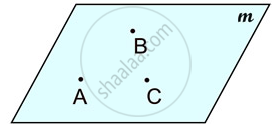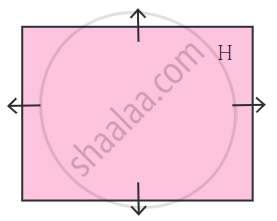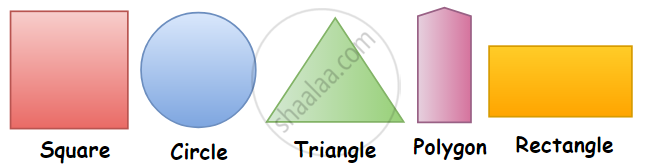# Concept of Plane

#### definition

Plane: Plane is a flat surface on which a straight line joining any two points on it would wholly lie.

# Plane:• In mathematics, a flat surface is called a plane.

• Each flat surface is a part of an infinite surface.

• Plane is a flat surface on which a straight line joining any two points on it would wholly lie.
• Even though we draw a suitably small figure of the plane, it actually extends infinitely on all sides. Arrows are drawn to show that the plane extends infinitely in all directions. However, these arrows are often omitted for the sake of convenience.• The 2-dimensional shapes or objects in geometry are flat plane figures that have two dimensions – length and width. Two-dimensional or 2-D shapes do not have any thickness and can be measured in only two faces.

A plane figure can be made of straight lines, curved lines, or both straight and curved lines. The circle, the square, the rectangle, the quadrilateral and the triangle are examples of plane figures.If you would like to contribute notes or other learning material, please submit them using the button below.